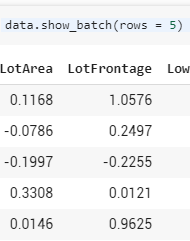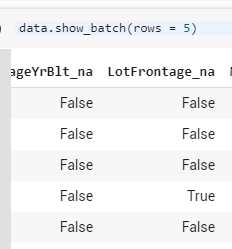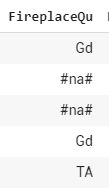# Why is my loss/error so big with this tabular learner?

I’m doing a regression problem with a datatset and I have a few questions. This is the book

1. I thought when I pass the procs such as fillmissing and categorize, it would change the values of the data, but nothing seems to be changed

2. Why is my error and loss rate so huge between the training set and validation set?

3. Why are my predicted values so small? Like the first value is -44.

Thanks!

FillMissing (if there are any) will generate a new #na# token whenever a value is missing, and a new column is generated with that variable _na. One example is your ‘LotFrontage’ variable. If we look at show-batch, we see the #na# value there, and the corresponding _na column for if the value was there or was not. I am curious as to why you chose to include “HalfBath” and other ‘numerical’ values as categorical. I’d associate the number of half-baths as a count rather than a category.

Categorify is passed into pandas itself, and essentially it is there to build the embedding matrix if you have categorical variables.

Second, if you look at the lesson 6 notebook, you can see that in order to get our actual predictions back from the regression notebook, we do:

``````test_preds=learn.get_preds(DatasetType.Test)
test_df["Sales"]=np.exp(test_preds.data).numpy().T
``````

Which I do not see something close to that in your notebook.

What you would do is:

``````preds, _ = learn.get_preds(ds_type=DatasetType.Test)
preds = np.exp(preds.data).numpy()
``````

Let me know if that changes anything. The notebook I am referring to is:

Hello! Thanks for replyAs for how FillMissing works, I think I understand now. However, looking at the values of lot frontageAll the values are there but the column for LotFrontage_na says True for the fourth row when it’s clearly not #na#Also, there’s other columns like Alley or Fence and FireplaceQu which look like thisBut there’s no FireplaceQu_na column.

To be honest I’m not exactly sure why I had those variables setup the way I did, but I think I seperated them properly now! However the loss’s are still very big.

I also fixed the last line to get the actual predictions but now most of the values seem to be infinity